# Thermal Storage and Release Features of Electric Thermal Storage Heating Systems with Solid Storage Material

Thermal Storage and Release Features of Electric Thermal Storage Heating Systems with Solid Storage Material
Hebei University of Architecture, Zhangjiakou 075024, China

Hebei University of Architecture, Zhangjiakou 075024, China

Corresponding Author Email:
syj@hebiace.edu.cn
Page:
1089-1098
|
DOI:
https://doi.org/10.18280/ijht.370418
12 May 2019
|
Accepted:
18 September 2019
|
Published:
26 December 2019
| Citation

OPEN ACCESS

Abstract:

This paper carries out simulation and tests on an electric thermal storage heating system with solid storage material (SS-ETSHS), and discusses the law of thermal storage and release in system operation, aiming to reduce the energy consumption and enhance the reliability and safety of the system. Based on the lumped parameter method, a mathematical model of the SS-ETSHS was established, and then solved by Matlab programming. The simulation results were used to analyze the change laws of the temperature of the thermal storage structure (TSS), circulating air temperature, and water temperature of the heat exchanger. The simulation results were proved valid and accurate through actual engineering tests. In addition, the patterns of temperature variation in different parts of the system were investigated under quantity adjustment and quality adjustment. The results show that the simulated results deviated from the measured results by 7.9% at the maximum, suggesting that our model and program are suitable for simulating the SS-ETSHS; in both adjustment modes, the residual heat of the TSS could not be released in the thermal release phase but built up, severely suppressing the thermal efficiency of the system. The research results are of great significance to the safe and reliable operation of the SS-ETSHS, shedding light on the optimization of the system.

Keywords:

electric thermal storage heating systems with solid storage material (SS-ETSHSs), lumped parameter method, features of thermal storage and release, quantity adjustment, quality adjustment

1. Introduction

Following the energy crisis in the 1970s, thermal storage has become a research hotspot in developed countries in Europe and North America. With energy-saving as the goal, the earliest thermal storage techniques mainly convert solar energy and wind energy into thermal energy, and gradually permeates to other industrial fields. After nearly five decades, foreign researchers have made marked achievements in the field of thermal storage. Many research outcomes have been applied widely in both civil and industrial fields. Since the 1990s, Chinese scholars began to design and manufacture equipment with solid thermal storage material, such as electric boilers. In recent years, China issued a series of policies to encourage energy saving and emission reduction and reduce off-peak electricity rate. As a result, regenerative electric heating systems have attracted growing attention from researchers and engineers. Meanwhile, the research into the following areas is gradually unfolded: the selection of thermal storage material, as well as the methods and features of thermal storage and release. The recent battle against smog raises new and higher requirements on the development and application of heat supply based on clean energy. Below is a brief review of thermal storage research at home and abroad.

Nordbeck et al.  proposed a modular cement based on the concept of solid-liquid thermal storage, and set up a test platform to examine the process and heat loss of thermal storage and release; the test results on temperature and heating rate were the same with the simulation data. Doppiu et al.  put forward an alternative material for thermal storage, analyzed different components theoretically, and measured the reversibility and stability of the material under experimental conditions. Esence et al.  compared the thermal performance between two regenerators, and evaluated their operating errors. Tian et al.  simulated the effective thermal conductivity of an autoclave aerated concrete (AAC). Ranjha et al. [5-10] studied the combination of different thermal storage materials and their heat storage capacities. Rao et al. [11-15] investigated the heat convection of the air on solid surfaces. Luo et al. [16-23] probed deep into the materials and process of thermal storage, and obtained the temperature distribution inside these materials.

To sum up, the existing studies mainly concentrate on the properties of thermal storage materials and thermal storage devices. There are relatively few reports on the operating features of electric thermal storage heating systems with solid storage material (SS-ETSHSs), not to mention the thermal storage and release features of the SS-ETSHSs in engineering applications. The application of the SS-ETSHSs still faces problems like unclear operation law, frequent system failures and high energy consumption.

Based on the principles of heat transfer and fluid mechanics, this paper establishes a mathematical model of an SS-ETSHS, and solves the model by Matlab programming. The thermal storage and release laws of the system were examined under changing external load. The research results provide technical supports to the actual operation of the SS-ETSHSs.

The remainder of this paper is organized as follows: Section 2 establishes the mathematical model of an SS-ETSHS; Section 3 solves the established model; Section 4 verifies the accuracy of the model through an engineering test; Section 5 analyzes the test results; Section 6 draws the conclusions.

2. Modelling

2.1 Physical model

As the heat source, the SS-ETSHS supplies hot water to the user for the purpose of heating. As shown in Figure 1, the SS-ETSHS mainly consists of the thermal storage structure (TSS), water pumps, heat exchangers, circulating fans, pipes, valves and a control system.

In the phase of thermal storage, the electric heating wire (EHW) in each vent is energized to heat up the TSS. Meanwhile, the circulating fans enter operation. Through convection, the air carries away some of the heat from the EHWs and the TSS, and then enters the heat exchangers to exchange heat with the water on the secondary side.

In the phase of thermal release, the power is turned off, and the TSS alone provides heat to the user.

## 1.pngFigure 1. The process flow of the SS-ETSHS

2.2 Construction of mathematical model

2.2.1 Hypotheses

Our research aims to disclose the change law of the temperature of the solid TSS with the outdoor temperature and operating time. The temperature distribution in the TSS was not considered. Therefore, the lumped parameter method was adopted for subsequent analysis. The following hypotheses were put forward:

(1) The solid TSS temperature is evenly distributed. The temperature distribution of the solid TSS at a moment is represented by the mean temperature Tx of the TSS at that moment.

(2) In the through-hole, the heat exchange between the air and the surfaces of the EHW and the TSS is not related to the flow state of the air; only the heat gain of the air is considered.

(3) Each heat exchanger is filled with pure water, with not phase change; the temperature distribution in the heat exchanger is represented by the mean temperature at the moment.

2.2.2 The thermal storage process

(1) Equations of the TSS

The variation of the thermal energy stored in the TSS with temperature can be described as:

$\mathrm{d} \Phi_{\mathrm{x}}=\operatorname{mcd} \mathrm{T}_{\mathrm{x}}$       (1)

where, $\Phi_{x}$ is thermal energy stored in the solid $\mathrm{TSS}, \mathrm{kj} ; \mathrm{m}$ is the mass of the solid TSS, kg; $c$ is the specific heat capacity of the solid TSS, kj $\cdot(\mathrm{kg} \cdot \mathrm{K})^{-1} ; \mathrm{T}_{\mathrm{x}}$ is the mean temperature of the solid TSS, K.

The amount of radiation of the EHW to the TSS can be expressed as:

$\mathrm{d} \Phi_{1}=\varepsilon \delta_{\mathrm{b}}\left(\mathrm{T}_{\mathrm{d}}^{4}-\mathrm{T}_{\mathrm{x}}^{4}\right) \mathrm{Ad} \tau$     (2)

where, $\Phi_{1}$ is the radiant heat received by the solid TSS, $\mathrm{kj}$; E is the absorption rate of the TSS; $\delta_{\mathrm{b}}$ is the Stefan-Boltzmann constant, $\delta_{\mathrm{b}}=5.67 \times 10^{-8} \mathrm{W} /\left(\mathrm{m}^{2} \cdot \mathrm{K}^{4}\right)$; $\mathrm{T}_{\mathrm{d}}$ is the surface temperature of the EHW, $\mathrm{K} ; \mathrm{T}_{\mathrm{x}}$ is the mean temperature of the solid TSS, K.

The amount of thermal conductivity of the EHW to the TSS can be expressed as:

$\mathrm{d} \Phi_{2}=\frac{2 \pi \lambda \mathrm{l}}{\ln \frac{\mathrm{r_0}}{\mathrm{r}_{1}}}\left(\mathrm{T}_{\mathrm{d}}-\mathrm{T}_{\mathrm{x}}\right) \mathrm{d} \tau$        (3)

where, $\Phi_{2}$ is the amount of thermal conductivity of the EHW to the solid TSS, $\mathrm{kj} ; \lambda$ is the conductivity coefficient of the solid TSS, W/( $\mathrm{m} \cdot \mathrm{K}) ; 1$ is the length of the through-hole of the solid TSS, $\mathrm{m} ; \mathrm{T}_{\mathrm{d}}$ is the surface temperature of the EHW, $\mathrm{K}$ $\mathrm{T}_{\mathrm{x}}$ is the mean temperature of the solid $\mathrm{TSS}, \mathrm{K} ; \mathrm{r}_{0}$ is radius of the through-hole in the solid TSS, $\mathrm{m} ; \mathrm{r}_{1}$ is the outer diameter of the through-hole in the solid TSS, m.

According to formulas (1), (2) and (3), the thermal storage of the TSS can be depicted as:

$\mathrm{mc} \frac{\mathrm{dT}_{\mathrm{x}}}{\mathrm{d} \tau}=\varepsilon \delta_{\mathrm{b}}\left(\mathrm{T}_{\mathrm{d}}^{4}-\mathrm{T}_{\mathrm{x}}^{4}\right) \mathrm{A}+\mathrm{n} \frac{2 \pi \lambda \mathrm{l}}{\mathrm{l} \mathrm{n} \frac{\mathrm{r}_{1}}{\mathrm{r}_{0}}}\left(\mathrm{T}_{\mathrm{d}}-\mathrm{T}_{\mathrm{x}}\right)$   (4)

(2) The heat transfer of the air in the solid TSS

In the thermal storage phase, the heat transfer of the air occurs simultaneously with the thermal storage in the solid TSS. The heat transfer of the air mainly exists as the convection on the surfaces of the EHW and the through-hole in the TSS.

By the lumped parameter method, the heat transfer accompanied by the air flow was ignored. Considering the large temperature difference between the air and the through-hole in the TSS, the convection coefficient can be calculated by the correlation recommended by Sieder and Tate. The correlation is applicable if Reynolds number Re>10,000, Prandtl number $\operatorname{Pr} \in[0.7,16,700]$, and length-to-diameter ratio L/di>60:

$\mathrm{Nu}_{\mathrm{f}}=0.023 \mathrm{Re}_{\mathrm{f}}^{0.8} \mathrm{Pr}_{\mathrm{f}}^{1 / 3}\left(\mathrm{T}_{\mathrm{f}} / \mathrm{T}_{\mathrm{w}}\right)^{0.55}$      (5)

where, $\mathrm{Nu}_{\mathrm{f}}$ is Nusselt number; $\operatorname{Re}_{\mathrm{f}}$ is Reynolds number; $\operatorname{Pr}_{\mathrm{f}}$ is Prandtl number; $\mathrm{T}_{\mathrm{f}}$ is the mean temperature of the air, $\mathrm{K} ; \mathrm{T}_{\mathrm{w}}$ is the wall temperature, K.

The Reynold number can be computed by:

$\operatorname{Re}_{\mathrm{f}}=\frac{\mathrm{d}_{\mathrm{i}} \mathrm{v} \rho}{\mu}$

where, $\mathrm{d}_{\mathrm{i}}$ is the diameter of through-hole, $\mathrm{m} ; \mathrm{v}$ is air velocity, $\mathrm{m} / \mathrm{s} ; \rho$ is air density, $\mathrm{kg} / \mathrm{m}^{3} ; \mu$ is hydrodynamic viscosity, $\mathrm{kg} /(\mathrm{m} \cdot \mathrm{s})$.

The Prandtl number can be computed by:

$\operatorname{Pr}_{\mathrm{f}}=\frac{\mathrm{c}_{\mathrm{p}} \mu}{\lambda_{\mathrm{a}}}$

where, $c_{p}$ is the isobaric specific heat capacity; $\lambda_{\mathrm{a}}$ is the thermal conductivity coefficient of the air, W/( $\mathrm{m} \cdot \mathrm{K})$.

According to Sieder and Tate’s correlation, the convection coefficient of the air in the circular through-hole can be described as:

$\mathrm{h}_{\mathrm{x}}=0.027 \frac{\lambda}{\mathrm{d}_{\mathrm{i}}}\left(\frac{\mathrm{d}_{\mathrm{i}} \mathrm{v} \rho}{\mu}\right)^{0.8}\left(\frac{\mathrm{c}_{\mathrm{p}} \mu}{\lambda}\right)^{1 / 3}\left(^{\mathrm{T}} \mathrm{f} / \mathrm{T}_{\mathrm{w}}\right)^{0.55}$

The heat transfer coefficient of the EHW to the air can be computed empirically by:

$\mathrm{h}_{\mathrm{d}}=2.25\left(\mathrm{T}_{\mathrm{d}}-\mathrm{T}_{\mathrm{a}}\right)^{0.25}+4.6 \times 10^{-8}\left(\mathrm{T}_{\mathrm{d}}^{2}+\mathrm{T}_{\mathrm{a}}^{2}\right)\left(\mathrm{T}_{\mathrm{d}}+\mathrm{T}_{\mathrm{a}}\right)$

The amount of heat convection between the air and the through-hole surface in the solid TSS can be computed by:

$\mathrm{d} \Phi_{\mathrm{x}}=\mathrm{h}_{\mathrm{x}} \mathrm{A}_{\mathrm{x}}\left(\mathrm{T}_{\mathrm{x}}-\mathrm{T}_{\mathrm{a}}\right) \mathrm{d} \tau$  (6)

The amount of heat exchange between the air and the EHW can be computed by:

$\mathrm{d} \Phi_{\mathrm{d}}=\mathrm{h}_{\mathrm{d}} \mathrm{A}_{\mathrm{d}}\left(\mathrm{T}_{\mathrm{d}}-\mathrm{T}_{\mathrm{a}}\right) \mathrm{d} \tau$        (7)

The heat gain of the air can be described as:

$\mathrm{d} \Phi_{\mathrm{a}}=\rho \mathrm{VC}_{\mathrm{p}} \mathrm{d} \mathrm{T}_{\mathrm{a}}$           (8)

According to formulas (5)-(8), we have:

$\rho \mathrm{VC}_{\mathrm{p}} \frac{\mathrm{dT}_{\mathrm{a}}}{\mathrm{d} \tau}=\mathrm{h}_{\mathrm{x}} \mathrm{A}_{\mathrm{x}}\left(\mathrm{T}_{\mathrm{x}}-\mathrm{T}_{\mathrm{a}}\right)+\mathrm{h}_{\mathrm{d}} \mathrm{A}_{\mathrm{d}}\left(\mathrm{T}_{\mathrm{d}}-\mathrm{T}_{\mathrm{a}}\right)$     (9)

(3) Heat equilibrium equation of heat exchanger

Using lumped parameter method, the heat equilibrium equation of heat exchanger can be established as follows:

The heat input from the air on the primary side can be described as:

$\mathrm{Q}_{\mathrm{in}}=\mathrm{K}_{\mathrm{a}} \mathrm{A}_{\mathrm{a}}\left(\mathrm{T}_{1}-\mathrm{T}_{2}\right)$       (10)

where, $\mathrm{K}_{\mathrm{a}}$ is the convection coefficient between the air and the inner wall of the heat exchanger, $\mathrm{W} /\left(\mathrm{m}^{2} \cdot \mathrm{K}\right) ; \mathrm{A}_{\mathrm{a}}$ is the contact area between the air and the heat exchanger, $\mathrm{m}^{2} ; \mathrm{T}_{1}$ is the inlet temperature of the air on the primary side, $K ; T_{2}$ is the outlet temperature of the air on the primary side, K.

Let the inlet temperature of heat user as the outlet temperature on the secondary side. Then, the heat output of the water on the secondary side can be illustrated as:

$\mathrm{Q}_{\mathrm{out}}=\mathrm{c}_{\mathrm{w}} \mathrm{q}_{\mathrm{w}}\left(\mathrm{T}_{3}-\mathrm{T}_{4}\right)$     (11)

where, $c_{w}$ is the specific heat capacity of water, $4.2 \times$ $10^{3} \mathrm{J} /(\mathrm{kg} \cdot \mathrm{K}) ; \mathrm{q}_{\mathrm{w}}$ is the flow of the secondary-side water, $\mathrm{m}^{3} / \mathrm{h} ; \mathrm{T}_{3}$ is the temperature of pure water in heat exchanger, $\mathrm{K} ; \mathrm{T}_{4}$ is the secondary-side outlet temperature of heat exchanger, K.

The net heat gain of the water in the heat exchange can be described as:

$\mathrm{d} \mathrm{Q}_{\mathrm{h}}=\mathrm{C}_{\mathrm{e}} \mathrm{d} \mathrm{T}_{3}$           (12)

The following can be derived from the heat equilibrium equation:

$\mathrm{d} \mathrm{Q}_{\mathrm{h}}=\mathrm{Q}_{\mathrm{in}} \mathrm{d} \tau-\mathrm{Q}_{\mathrm{out}} \mathrm{d} \tau$       (13)

According to formulas (10)-(13), we have:

$\mathrm{C}_{\mathrm{e}} \frac{\mathrm{dT}_{3}}{\mathrm{d} \tau}=\mathrm{K}_{\mathrm{a}} \mathrm{A}_{\mathrm{a}}\left(\mathrm{T}_{1}-\mathrm{T}_{2}\right)-\mathrm{c}_{\mathrm{w}} \mathrm{q}_{\mathrm{w}}\left(\mathrm{T}_{3}-\mathrm{T}_{4}\right)$     (14)

2.2.3 The thermal release phase

(1) The variation of the thermal energy stored in the TSS with temperature can be described as:

$\mathrm{d} \Phi_{\mathrm{x}}=\operatorname{mcd} \mathrm{T}_{\mathrm{x}}$       (15)

Where, $\Phi_{x}$ is thermal energy stored in the solid $\mathrm{TSS}, \mathrm{kj} ; \mathrm{m}$ is the mass of the solid TSS, kg; $c$ is the specific heat capacity of the solid TSS, kj $\cdot(\mathrm{kg} \cdot \mathrm{K})^{-1} ; \mathrm{T}_{\mathrm{x}}$ is the mean temperature of the solid TSS, K.

The amount of the heat exchange between the TSS and the air can be expressed as:

$\left\{\begin{array}{c}{\mathrm{d} \Phi_{\mathrm{h}}=\alpha_{\mathrm{a}}\left(\mathrm{T}_{\mathrm{a}}-\mathrm{T}_{\mathrm{x}}\right) \mathrm{d} \tau} \\ {\alpha_{\mathrm{a}}=\alpha_{1}+\alpha_{2}}\end{array}\right.$          (16)

where, $\Phi_{4}$ is the amount of thermal conductivity of solid TSS to the air, $\mathrm{kj} ; \alpha_{\mathrm{a}}$ is the synthetic heat transfer coefficient of solid TSS to the air, $\mathrm{W} /(\mathrm{m} \cdot \mathrm{K}) ; \alpha_{1}$ is the radiation coefficient of solid TSS to the air, W/( $\mathrm{m} \cdot \mathrm{K}) ; \alpha_{2}$ is the convection coefficient of solid TSS to the air, W/( $\mathrm{m} \cdot \mathrm{K}) ; \mathrm{T}_{\mathrm{X}}$ is the mean temperature of solid TSS, K; $T_a$ is the mean temperature of the } air, K.

According to formulas (15) and (16), the thermal release equation of the TSS can be obtained as:

$\mathrm{mc} \frac{\mathrm{dT}_{\mathrm{x}}}{\mathrm{d\tau}}=\alpha_{\mathrm{a}}\left(\mathrm{T}_{\mathrm{a}}-\mathrm{T}_{\mathrm{x}}\right)$       (17)

(2) The heat transfer of the air in the solid TSS

Considering the large temperature difference between the air and the through-hole in the TSS, the heat transfer of the air in the solid TSS can be calculated by the correlation recommended by Sieder and Tate. The correlation is applicable if Reynolds number Re>10,000, Prandtl number Pr∈[0.7, 16,700], and length-to-diameter ratio L/di>60:

$\mathrm{Nu}_{\mathrm{f}}=0.027 \mathrm{Re}_{\mathrm{f}}^{0.8} \mathrm{Pr}_{\mathrm{f}}^{1 / 3}\left(\mathrm{T}_{\mathrm{f}} / \mathrm{T}_{\mathrm{w}}\right)^{0.55}$      (18)

where, $\mathrm{Nu}_{\mathrm{f}}$ is Nusselt number; $\operatorname{Re}_{\mathrm{f}}$ is Reynolds number; $\operatorname{Pr}_{\mathrm{f}}$ is Prandtl number; $\mathrm{T}_{\mathrm{f}}$ is the mean temperature of the air, $\mathrm{K} ; \mathrm{T}_{\mathrm{w}}$ is the wall temperature, K.

The Reynold number can be computed by:

$\operatorname{Re}_{\mathrm{f}}=\frac{\mathrm{d}_{\mathrm{i}} \mathrm{v} \rho}{\mu}$

where, $\mathrm{d}_{\mathrm{i}}$ is the diameter of through-hole, $\mathrm{m} ; \mathrm{v}$ is air velocity, $\mathrm{m} / \mathrm{s} ; \rho$ is air density, $\mathrm{kg} / \mathrm{m}^{3} ; \mu$ is hydrodynamic viscosity, $\mathrm{kg} /(\mathrm{m} \cdot \mathrm{s})$

The Prandtl number can be computed by:

$\operatorname{Pr}_{\mathrm{f}}=\frac{\mathrm{c}_{\mathrm{p}} \mu}{\lambda_{\mathrm{a}}}$

where, $c_{p}$ is the isobaric specific heat capacity; $\lambda_{a}$ is the thermal conductivity coefficient of the air, W/( $\mathrm{m} \cdot \mathrm{K})$.

According to Sieder and Tate’s correlation, the convection coefficient of the air in the circular through-hole can be described as:

$\mathrm{h}_{\mathrm{x}}=0.027 \frac{\lambda}{\mathrm{d}_{\mathrm{i}}}\left(\frac{\mathrm{d}_{\mathrm{i}} \mathrm{v} \rho}{\mu}\right)^{0.8}\left(\frac{\mathrm{c}_{\mathrm{p}} \mu}{\lambda}\right)^{0.4}\left(\mathrm{T}_{\mathrm{f}} / \mathrm{T}_{\mathrm{w}}\right)^{0.55}$

The heat gain of the air through radiation can be described as:

$\mathrm{d} \Phi_{\mathrm{a}}=\varepsilon \delta_{\mathrm{b}}\left(\mathrm{T}_{\mathrm{a}}^{4}-\mathrm{T}_{\mathrm{x}}^{4}\right) \mathrm{Ad} \tau$      (19)

where, $\Phi_{\mathrm{a}}$ is the radiant heat of the solid TSS to the air, $\mathrm{kj} ; \varepsilon$ is the absorption rate of the air; $\delta_{b}$ is the Stefan-Boltzmann constant, $\delta_{\mathrm{b}}=5.67 \times 10^{-8} \mathrm{W} /\left(\mathrm{m}^{2} \cdot \mathrm{K}^{4}\right) ; \mathrm{T}_{\mathrm{x}}$ is the mean temperature of the solid TSS, K; $T_a$ is the mean temperature of } the air, K.

According to formulas (18) and (19), we have:

$\rho \mathrm{VC}_{\mathrm{p}} \frac{\mathrm{dT}_{\mathrm{a}}}{\mathrm{d} \tau}=\mathrm{h}_{\mathrm{x}} \mathrm{A}\left(\mathrm{T}_{\mathrm{x}}-\mathrm{T}_{\mathrm{a}}\right)+\varepsilon \delta_{\mathrm{b}}\left(\mathrm{T}_{\mathrm{x}}^{4}-\mathrm{T}_{\mathrm{a}}^{4}\right) \mathrm{A}$     (20)

(3) Heat equilibrium equation of heat exchanger

The heat input from the air on the primary side can be described as:

$\mathrm{Q}_{\mathrm{in}}=\mathrm{K}_{\mathrm{a}} \mathrm{A}_{\mathrm{a}}\left(\mathrm{T}_{1}-\mathrm{T}_{2}\right)$       (21)

where, $\mathrm{K}_{\mathrm{a}}$ is the convection coefficient between the air and the inner wall of the heat exchanger, $\mathrm{W} /\left(\mathrm{m}^{2} \cdot \mathrm{K}\right) ; \mathrm{A}_{\mathrm{a}}$ is the contact area between the air and the heat exchanger, $\mathrm{m}^{2} ; \mathrm{T}_{1}$ is the inlet temperature of the air on the primary side, $K ; T_{2}$ is the outlet temperature of the air on the primary side, K.

Let the inlet temperature of heat user as the outlet temperature on the secondary side. Then, the heat output of the water on the secondary side can be illustrated as:

$\mathrm{Q}_{\mathrm{out}}=\mathrm{c}_{\mathrm{w}} \mathrm{q}_{\mathrm{w}}\left(\mathrm{T}_{3}-\mathrm{T}_{4}\right)$           (22)

where, $c_{w}$ is the specific heat capacity of water, $4.2 \times$ $10^{3} \mathrm{J} /(\mathrm{kg} \cdot \mathrm{K}) ; \mathrm{q}_{\mathrm{w}}$ is the flow of the secondary-side water, $\mathrm{m}^{3} / \mathrm{h} ; \mathrm{T}_{3}$ is the temperature of pure water in heat exchanger, $\mathrm{K} ; \mathrm{T}_{4}$ is the secondary-side outlet temperature of heat exchanger, K.

The net heat gain of the water in the heat exchange can be described as:

$\mathrm{d} \mathrm{Q}_{\mathrm{h}}=\mathrm{C}_{\mathrm{e}} \mathrm{d} \mathrm{T}_{3}$            (23)

The following can be derived from the heat equilibrium equation:

$\mathrm{dQ}_{\mathrm{h}}=\mathrm{Q}_{\mathrm{in}} \mathrm{d} \tau-\mathrm{Q}_{\mathrm{out}} \mathrm{d} \tau$             (24)

According to formulas (21)-(24), we have:

$\mathrm{C}_{\mathrm{e}} \frac{\mathrm{dT}_{3}}{\mathrm{d} \tau}=\mathrm{K}_{\mathrm{a}} \mathrm{A}_{\mathrm{a}}\left(\mathrm{T}_{2}-\mathrm{T}_{1}\right)-\mathrm{c}_{\mathrm{w}} \mathrm{q}_{\mathrm{w}}\left(\mathrm{T}_{3}-\mathrm{T}_{4}\right)$      (25)

3. Model Solving

The above equations were solved by Matlab programming to reveal the law of thermal storage and release. For the thermal storage model, the physical parameters of the solid TSS, the radiation coefficient and thermal conductivity coefficient are all known. According to the manufacturer’s data, the EHW can be heated up quickly to 900-1,100℃. The mean temperature, 1,000℃, of the EHW was adopted for our calculation. The convection coefficients of the air to the EHW and the TSS are both known. The parameters of the heat exchanger and the flow of the water pump were obtained through calculation. During the calculation, the Tx value obtained by formula (4) was substituted into formula (9), and the result of formula (9) was then substituted to formula (14). The air temperature at the inlet of the heat exchanger was considered as the air temperature Ta. The temperature of the return air was set empirically to 60℃. The return water temperature of the pump was calculated according to the design temperature. The solving process of the thermal storage model is explained in Figure 2. The thermal release model was solved in a similar manner.

## 2.jpgFigure 2. Solving process of the thermal storage model

4. Test Verification

4.1 Test device

The established model was verified through a test on a 1.5MW SS-ETSHS, which can supply heat to 20,000m2 of residential buildings. The terminals of the system are finned radiators. The system uses 10kV high-voltage electricity for heating. The thermal storage period and thermal release period are both 120min. In the thermal storage phase, the system supplies heat to users while storing thermal energy; in the thermal release phase, the heat in the TSS is released to heat up the users.

For the safety and efficiency of thermal storage, the TSS is divided into three parts, each of which is provided with a 10,000m³/h variable frequency fan and a 0.5MW steam-water heat exchanger.

The physical parameters of the TSS are as follows: the maximum designed temperature for thermal storage, 700℃; external dimensions, 3.9m in length, 1.9m in width and 1.1m in height; vent dimensions, 40mm in diameter, 1.9m in depth; number of vents, 14 in the horizontal direction, 18 in the vertical direction; insulation layer, special insulation cotton; shell, 50cm in thickness.

4.2 Test instruments

The TSS temperature and hot air temperature were measured by nickel-chromium/nickel-silicon type K thermocouples (accuracy: grade 1; measuring range: 0-1,300℃). The water temperature was measured by a PT100 temperature sensor (accuracy: grade 1; measuring range: 0-150℃). The outdoor temperature was measured by a PT100 temperature sensor (accuracy: grade 1; measuring range: -50-50℃).

4.3 Test plan

TSS temperature was measured through contact testing by the thermocouples. One thermocouple was placed at 0.6m ahead of the inlet of the TSS, and another at 0.6m after the outlet of the TSS. Two thermocouples were deployed respectively at the inlet and the outlet of each vent to capture the inlet and outlet air temperatures. Temperature sensors were arranged in the heat exchanger, the inlet of the water pump and the outlet of the water pump. The temperatures collected from different positions were all displayed on the monitor.

5. Test Results and Analysis

5.1 Reliability of model and program

The measured data were compared with the simulated data to verify the reliability of our model and program. Figures 3-5 show how the temperature of the TSS, the primary-side air and the heat exchanger vary with time in the thermal storage phase, respectively. Figures 6-8 present how the temperature of the TSS, the primary-side air and the heat exchanger vary with time in the thermal release phase.

It can be seen that, in the thermal storage phase, the test data on the TSS temperature, the air temperature and the heat exchanger temperature had basically the same trends with the simulated data, with the peak error of 7.9%; in the thermal release phase, the test data generally agree with the simulated data, with the peak error of 7.1%. The results show that our model and program are suitable for the research on the law of thermal storage and release.

## 3.jpgFigure 3. Time variation in TSS temperature

## 4.jpg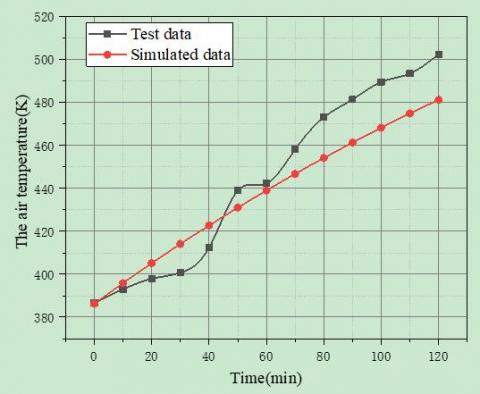Figure 4. Time variation in air temperature

## 5.jpg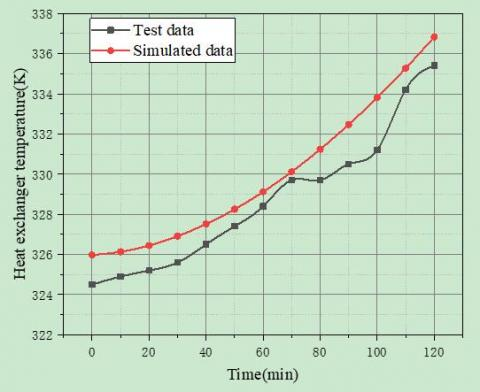Figure 5. Time variation in heat exchanger temperature

## 6.jpg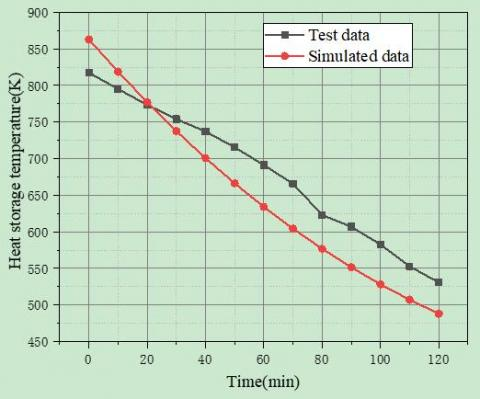Figure 6. Time variation in TSS temperature

## 7.jpg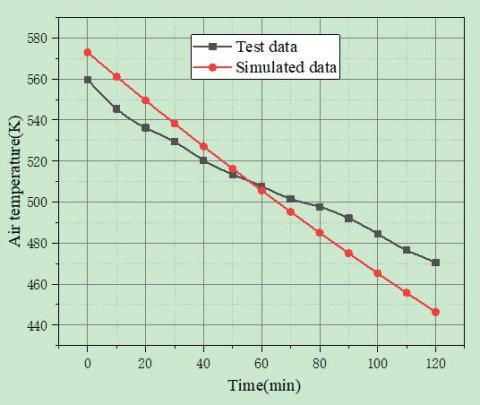Figure 7. Time variation in air temperature

## 8.jpg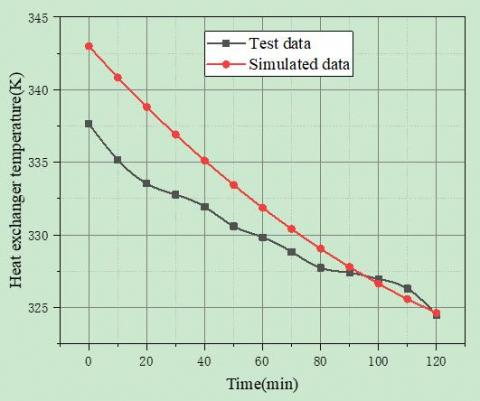Figure 8. Time variation in heat exchanger temperature

5.2 Simulation of heat storage and release features

The operating features of the SS-ETSHS were investigated through the quantity adjustment of the external heat supply system, i.e. changing the frequency of each circulating water pump without changing the water supply temperature.

## 9.jpgFigure 9. Time variation of TSS temperature in thermal storage phase

## 10.jpgFigure 10. Time variation of heating rate of the TSS

Figures 9 and 10 describe the time variation of TSS temperature in thermal storage phase and that of heating rate of the TSS, respectively.

As shown in Figure 9, in the heat storage phase, the TSS temperature increased gradually with the elapse of time, from 500K to 900K in 120min. Meanwhile, the external heat output increased with the frequency of the circulating water pump. As a result, the TSS temperature dropped from the 900K at the 100% pump frequency to the 830K at 70% pump frequency. The falling TSS temperature occurred because the growing external output load pushes up the heat output of the primary-side air.

As shown in Figure 10, the heating rate of the solid TSS decreased with the rising temperature of the TSS. This is because the TSS temperature gradually approaches the EHW temperature in the heating process, and the heating rate gradually decreases with the temperature transfer rate inside the TSS. Despite the adjustment of pump frequency, the TSS heating rate was basically stable. The heating rate at 70% pump frequency was about 1.2 times that at 100% pump frequency.

## 11.jpgFigure 11. Time variation of TSS temperature in thermal release phase

## 12.jpgFigure 12. Time variation of cooling rate of the TSS

Figures 11 and 12 depict the time variation of TSS temperature in thermal storage phase and that of cooling rate of the TSS, respectively.

As shown in Figure 11, the TSS temperature always decreased at different pump frequency, from 900K to 610K in 120min. With the decline of pump frequency, the TSS temperature continued to drop, and the maximum temperature drop was 130K.

As shown in Figure 12, the cooling rate of the TSS varied with the pump frequencies. After adjusting the pump frequency, the operator should finetune the fans to satisfy the heat demand on the secondary side. After 90min of thermal release, the cooling rate curves at different pump frequencies intersected at one point, indicating that the pump flow increased and TSS temperature dropped faster after 90min. The TSS was cooled down rapidly at 100% pump frequency. At 90min, the TSS temperature fell to 500K. In this case, the TSS can no longer release heat, failing to satisfy the heating demand.

The operating features of the SS-ETSHS were further investigated through the quality adjustment of the external heat supply system, i.e. changing the water temperature without changing the flow of water supply.

During quality adjustment, the frequency of the circulating water pump on the secondary side was set to 70%. The temperature of water supply was regulated by changing the operating state of the fans. The shift from quantity adjustment to quality adjustment only changes the secondary-side flow or the temperature of incoming and return water, which has little impact on the TSS. Hence, the author only simulated how the air temperature and heat exchanger temperature varied during the quality adjustment.

## 13.jpgFigure 13. Time variation of air temperature in thermal storage phase

## 14.jpgFigure 14. Time variation of heat exchanger temperature in thermal storage phase

Figures 13 and 14 show the time variation of air temperature and heat exchanger temperature in thermal storage phase, respectively.

As shown in Figure 13, the outlet air temperature gradually rose at different fan frequencies. When the fans operated at the rated frequency of 100%, the outlet air temperature peaked at 465K.

As shown in Figure 14, the heat exchanger temperature increased over time in the thermal storage phase, changing the water supply temperature on the secondary side. In addition, the heat exchanger temperature rose continuously with the growing frequency of fans.

## 15.jpg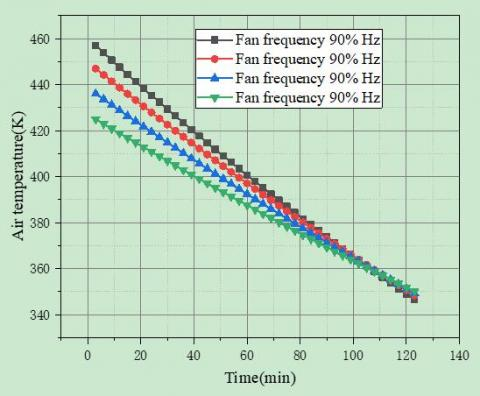Figure 15. Time variation of air temperature in thermal release phase

## 16.jpgFigure 16. Time variation of heat exchanger temperature in thermal release phase

Figures 15 and 16 show the time variation of air temperature and heat exchanger temperature in thermal release phase, respectively.

As shown in Figure 15, the air temperature curves at different fan frequencies converged at a point at 100min into the thermal release. A possible reason is that the air releases heat slower than the TSS. At 100min, the heat provided by the air cannot fulfill the heating demand.

As shown in Figure 16, the heat exchanger temperatures at different fan frequencies were all decreasing over the time, and became basically constant after about 100min. At this moment, the heat exchanger temperature is basically stable, outputting no heat to the secondary side.

6. Conclusions

The SS-ETSHS is a suitable heat supply strategy for the Coal-to-Electricity Project in China. This paper explores the thermal storage and release features of the system through simulation and tests.

During the quantity adjustment of the secondary side, the following phenomena were observed in the thermal storage phase: the TSS temperature increased over time, peaking at 900K; the heating rate gradually decreased with the temperature difference. Meanwhile, the following phenomena were observed in the thermal release phase: the TSS temperature decreased over time, minimizing at 500K; the cooling rate exhibited a gradual decline, yet the declining rate varied with the frequency of the circulating water pump; the smaller the pump frequency, the slower the trend of decline; the cooling rate curves intersected each other at about 90min, indicating that the TSS cooling speeds up due to the growth in water flow.

During the quality adjustment of the secondary side, the following phenomena were observed in the thermal storage phase: the TSS temperature gradually rose with the elapse of time, but the heating rate was smaller than the quantity adjustment mode. Meanwhile, the following phenomena were observed in the thermal release phase: the air temperature curves at different fan frequencies converged at one point at 100min into the heat release. A possible reason is that the air releases heat slower than the TSS. At 100min, the heat provided by the air cannot fulfill the heating demand.

In both adjustment modes, the TSS could not release the residual heat at the end of the thermal release phase. The residual heat of the TSS simply built up, severely suppressing the thermal efficiency of the system.

Acknowledgments

This work is supported by Hebei innovation capability improvement project (19244503D).

References

 Nordbeck, J., Bauer, S., Beyer, C. (2019). Experimental characterization of a lab-scale cement based thermal energy storage system. Applied Energy, 256: 113937. https://doi.org/10.1016/j.apenergy.2019.113937

 Doppiu, S., Dauvergne, J.L., Palomo del Barrio, E. (2019). Solid-state reactions for the storage of thermal energy. Nanomaterials, 9(2): 226. https://doi.org/10.3390/nano9020226

 Esence, T., Desrues, T., Fourmigué, J.F., Cwicklinski, G., Bruch, A., Stutz, B. (2019). Experimental study and numerical modelling of high temperature gas/solid packed-bed heat storage systems. Energy, 180: 61-78. https://doi.org/10.1016/j.energy.2019.05.012

 Tian, S.Q., Yu, S.F., Wang, X., Fan, L.W., Yu, Z.T., Xu, X., Ge, J. (2019). Experimental determination and fractal modeling of the effective thermal conductivity of autoclave aerated concrete (AAC) impregnated with paraffin for improved thermal storage performance. Applied Thermal Engineering, 163: 114387. https://doi.org/10.1016/j.applthermaleng.2019.114387

 Ranjha, Q., Vahedi, N., Oztekin, A. (2019). High-temperature thermochemical energy storage–heat transfer enhancements within reaction bed. Applied Thermal Engineering, 163: 114407. https://doi.org/10.1016/j.applthermaleng.2019.114407

 Beemkumar, N., Karthikeyan, A., Ramachandran, S. (2015). Heat transfer enhancement of the latent heat storage system using different encapsulating materials with and without fins. International Journal of Ambient Energy, 38(1): 77–84. https://doi.org/10.1080/01430750.2015.1035801

 Kaggwa, A., Carson, J.K. (2019). Developments and future insights of using nanofluids for heat transfer enhancements in thermal systems: A review of recent literature. International Nano Letters, 9(4): 277-288. https://doi.org/10.1007/s40089-019-00281-x

 Khan, Z., Khan, Z.A., Sewell, P. (2019). Heat transfer evaluation of metal oxides based nano-PCMs for latent heat storage system application. International Journal of Heat and Mass Transfer, 144: 118619. https://doi.org/10.1016/j.ijheatmasstransfer.2019.118619

 Siahpush, A., O'Brien, J., Crepeau, J., Sabharwall, P. (2019). Experimental and scale analysis of a solid/liquid phase change thermal energy storage system. Heat Transfer Engineering, 40(19): 1600-1618. https://doi.org/10.1080/01457632.2018.1480878

 Saranprabhu, M.K., Rajan, K.S. (2019). Enhancement of solid-phase thermal conductivity and specific heat of solar salt through addition of MWCNT: New observations and implications for thermal energy storage. Applied Nanoscience, 9(8): 2117-2126. https://doi.org/10.1007/s13204-019-01107-0

 Rao, C.R.C., Vigneshwaran, K., Niyas, H., Muthukumar, P. (2019). Performance investigation of lab-scale sensible heat storage prototypes. International Journal of Green Energy, 16(14): 1363-1378. https://doi.org/10.1080/15435075.2019.1671411

 Kunkel, S., Teumer, T., Dörnhofer, P., Schlachter, K., Weldeslasie, Y., Kühr, M., Repke, J.U. (2018). Determination of heat transfer coefficients in direct contact latent heat storage systems. Applied Thermal Engineering, 145: 71-79. https://doi.org/10.1016/j.applthermaleng.2018.09.015

 André, L., Abanades, S., Cassayre, L. (2018). Mixed metal oxide systems applied to thermochemical storage of solar energy: Benefits of secondary metal addition in Co and Mn oxides and contribution of thermodynamics. Applied Sciences, 8(12): 2618. https://doi.org/10.3390/app8122618

 Arena, S., Casti, E., Gasia, J., Cabeza, L.F., Cau, G. (2018). Numerical analysis of a latent heat thermal energy storage system under partial load operating conditions. Renewable Energy, 128: 350-361. https://doi.org/10.1016/j.renene.2018.05.072

 Tabassum, T., Hasan, M., Begum, L. (2018). Dynamic heat transfer study of a triangular-shaped latent heat storage unit for the attic space of a domestic dwelling. Journal of Thermal Science and Engineering Applications, 10(6): 061015. https://doi.org/10.1115/1.4040645

 Luo, X., Liao, S. (2018). Numerical study on melting heat transfer in dendritic heat exchangers. Energies, 11(10): 2504. https://doi.org/10.3390/en11102504

 Mahdi, M.A., Smaili, A., Saad, Y. (2020). Numerical investigations of turbulent natural convection heat transfer within a wind turbine nacelle operating in hot climate. International Journal of Thermal Sciences, 147: 106143. https://doi.org/10.1016/j.ijthermalsci.2019.106143

 Gui, X., Song, X., Cheng, L., Jia, W., Nie, B., Xu, G. (2019). Influence of leakage of air hole on flow and heat transfer in recuperator. Energy Sources, Part A: Recovery, Utilization, and Environmental Effects, 1-17. https://doi.org/10.1080/15567036.2019.1668886

 Wang, C., Mobedi, M., Kuwahara, F. (2019). Simulation of heat transfer in a closed-cell porous media under local thermal non-equilibrium condition. International Journal of Numerical Methods for Heat & Fluid Flow, 29(8): 2478-2500. https://doi.org/10.1108/HFF-01-2019-0081

 Royo, P., Acevedo, L., Ferreira, V. J., García-Armingol, T., López-Sabirón, A.M., Ferreira, G. (2019). High-temperature PCM-based thermal energy storage for industrial furnaces installed in energy-intensive industries. Energy, 173: 1030-1040. https://doi.org/10.1016/j.energy.2019.02.118

 Sheikholeslami, M., Haq, R.U., Shafee, A., Li, Z., Elaraki, Y.G., Tlili, I. (2019). Heat transfer simulation of heat storage unit with nanoparticles and fins through a heat exchanger. International Journal of Heat and Mass Transfer, 135: 470-478. https://doi.org/10.1016/j.ijheatmasstransfer.2019.02.003

 Wang, Z.W., Lv, C.N., Cai, J.L., Qian, L., Li, Y.Y. (2018). Optimization design of high temperature solid heat storage system for photo thermal power generation. Energy Conservation Technology, 36(4): 357-360. https://doi.org/10.3969/j.issn.1002-6339.2018.04.013

 Zhang, N., Yuan, Y., Cao, X., Du, Y., Zhang, Z., Gui, Y. (2018). Latent heat thermal energy storage systems with solid–liquid phase change materials: A review. Advanced Engineering Materials, 20(6): 1700753. https://doi.org/10.1002/adem.201700753